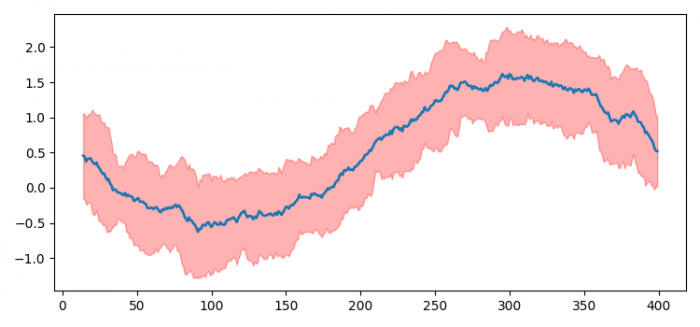# How to plot a time series array, with confidence intervals displayed in Python? (Matplotlib)

To plot a time series array, with confidence intervals displayed in Python, we can take the following steps −

• Set the figure size and adjust the padding between and around the subplots.
• Get the time series array.
• Initialize a variable, n_steps, to get the mean and standard deviation.
• Get the under and above lines for confidence intervals.
• Plot the mean line using plot() method.
• Use fill_between() method to get the confidence interval.
• To display the figure, use show() method.

## Example

import numpy as np
import pandas as pd
import matplotlib.pyplot as plt

plt.rcParams["figure.figsize"] = [7.50, 3.50]
plt.rcParams["figure.autolayout"] = True

time_series_array = np.sin(np.linspace
(-np.pi, np.pi, 400)) + np.random.rand((400))
n_steps = 15

time_series_df = pd.DataFrame(time_series_array)

line = time_series_df.rolling(n_steps).mean()

line_deviation = 2 * time_series_df.rolling(n_steps).std()

under_line = (line - line_deviation)

over_line = (line + line_deviation)

plt.plot(line, linewidth=2)

plt.fill_between(line_deviation.index, under_line,
over_line, color='red', alpha=.3)

plt.show()

## Output